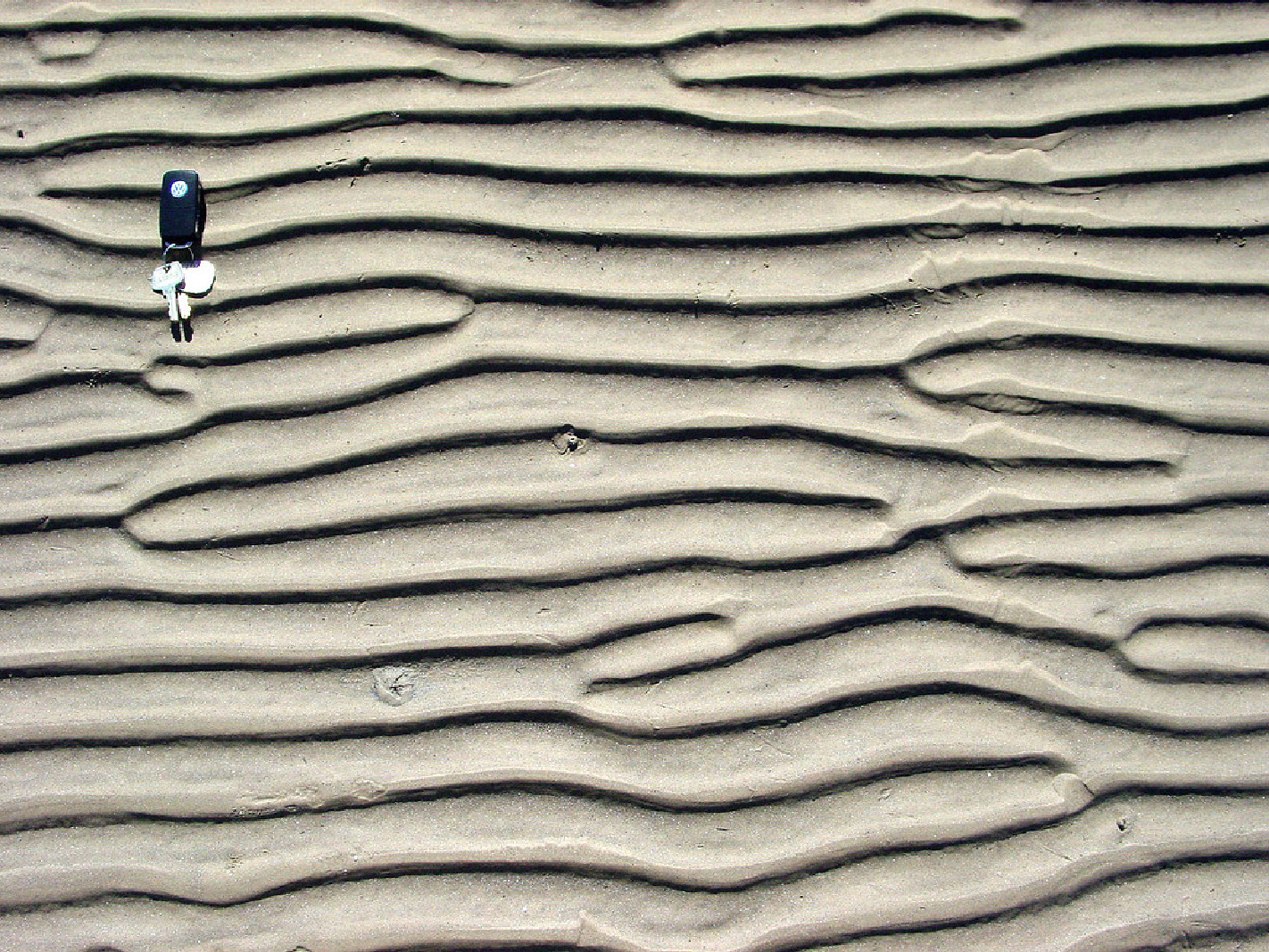# Modelling wind ripples

Here, I try to simulate the patterns obtained on a sandy terrain. This is what is observed when the wind blows on the dry surface of a land with sand or also on the surface of the sea in the presence of currents.

As described in https://en.wikipedia.org/wiki/Dune,

When a sandy seabed is subject to wave action and the wave orbital motion is strong enough to move sand grains, ripples often appear. The ripples induced by wave action are called “wave ripples”; their characteristics being different from those of the ripples generated by steady flows. The most striking difference between wave ripple fields and current ripple fields is the regularity of the former. Indeed, regular long-crested wave ripple fields are often observed on tidal beaches from which the sea has withdrawn at low water (see figure 1).

A nice example is shown in http://www.coastalwiki.org/wiki/Wave_ripple_formation showing

Ripples observed at Sea Rim State Park, along the coast of east Texas close to the border with Louisiana (courtesy by Zoltan Sylvester).Wave ripple formation

An interesting aspect of that patterns is that they may occur at different scales, like taht example on the surface of the Mars planet:

Overview of large sand wave field and high-resolution difference map of two surveys approximately 21 hours apart illustrating both large-scale and small-scale sand wave migration and orientation. Migration is from right to left.Let's first initialize the notebook:

In :
import matplotlib
import numpy as np
np.set_printoptions(precision=6, suppress=True)
import os
%matplotlib inline
%config InlineBackend.figure_format='retina'
# %config InlineBackend.figure_format = 'svg'
import matplotlib.pyplot as plt
import cmocean # https://matplotlib.org/cmocean/
opts = dict(cmap=cmocean.cm.deep, interpolation='lanczos')
fig_width = 13
subplotpars = matplotlib.figure.SubplotParams(left=0., right=1., bottom=0., top=1., hspace=0., wspace=0.05,)

In :
# Importing libraries
import torch
import torch.nn.functional as nnf
torch.manual_seed(1998)
torch.set_printoptions(precision=3, linewidth=140, sci_mode=False)

if torch.backends.mps.is_available():
device = torch.device('mps')
elif torch.cuda.is_available():
device = torch.device('cuda')
else:
device = torch.device('cpu')

torch.__version__, device

Out:
('2.0.0', device(type='mps'))

### Wave & wind ripples¶

Such ripples can be charcterized using the physical process that generate them:

Sea waves shape the bottom and generate different morphological patterns, which are characterized by a wide range of length scales. The ripples are the smallest bedforms but, notwithstanding their relatively small size, they play a prominent role in many transport processes. Indeed, usually, the flow separates at their crests and vortices are generated which increase momentum transfer, sediment transport and, in general, mixing phenomena. (from http://www.coastalwiki.org/wiki/Wave_ripples)

And can be formalized using simple iterative process like that described in http://nishitalab.org/user/nis/cdrom/pg/onoue.pdf or a more complex example shown in https://www.hindawi.com/journals/jam/2014/590358/

We will try to give a simple formulation and first initialize the parameters:

In :
from dataclasses import dataclass, asdict
DEBUG = 2
DEBUG = 1

@dataclass
class Params:
bins: int = 2**(8//DEBUG)
N_particles: int = 2**(8*2) * 8
N_step: int = 2**(10//DEBUG)
L: float = 1 / 2**6 # saltation step in the y direction
kappa: float = 4.5
D: float = 8.e-4 # diffusion per step
seed : int = 1998

p = Params()
p

Out:
Params(bins=256, N_particles=524288, N_step=1024, L=0.015625, kappa=4.5, D=0.0008, seed=1998)

The dynamical system can be formalized by the following equations.

Diffusion step with $\nu$ a normal noise: $$\vec{x}_i \leftarrow \vec{x}_i + D \cdot \nu$$ where $\vec{x}_i = (x_i, y_i)$ corresponds to the position of the $i$-th particle.

We may compute the local height as the density of particle: $$h(\vec{x}) = \# \{ \vec{x}_i=\vec{x} | 1 \le i \le N \}$$ This is in practice defined on a local grid and with a finite number $N$ of particles.

We may finally define a saltation step in the wind direction direction: $$y_i \leftarrow y_i + L \cdot \sigma( - \kappa * h(\vec{x}))$$ where $\sigma$ is the sigmoid function $\sigma(x) = \frac 1 {1 + \exp(-x)}$. For computational tractability, coordinates are finally remapped on the torus.

Interestingly, this type of model combining a linear diffusion and a non-linear scaling is found in many different physical systems, including neural systems (random recurrent neural fields for instance) and has a long history in computational neuroscience. The implementation we chose is slightly different as the field is defined by particles, reminiscent of our previous work on modelling motion detection in the visual system.

The dynamical system can be simplified in the following code which models the terrain by sand particles and then estimates the terrain using a spatial histogram. First, we code it in numpy:

In :
def sigmoid(x):
return 1 / (1 + np.exp(-x))

def wind_ripples(p):

binz = [np.linspace(0, 1, p.bins+1, endpoint=True), np.linspace(0, 1, p.bins+1, endpoint=True)]
np.random.seed(p.seed)
x, y = np.random.rand(p.N_particles), np.random.rand(p.N_particles)

for i_step in range(p.N_step):

x, y = x + p.D/np.sqrt(i_step+1)*np.random.randn(p.N_particles), y + p.D/np.sqrt(i_step+1)*np.random.randn(p.N_particles)
x, y = x % 1, y % 1

height, edge_x, edge_y = np.histogram2d(x, y, bins=binz, density=True)

ind_x, ind_y = (x*p.bins).astype(int), (y*p.bins).astype(int)

y += p.L * sigmoid( - p.kappa * height[ind_x, ind_y])

return height


Which runs for N_step=2**7 on the CPU in about 12s:

In :
%timeit -n1 -r1 wind_ripples(Params(N_step=2**7))

12 s ± 0 ns per loop (mean ± std. dev. of 1 run, 1 loop each)


Then using pyTorch:

In :
def wind_ripples(p):
binz = (torch.linspace(0, 1, p.bins+1), torch.linspace(0, 1, p.bins+1))

np.random.seed(p.seed)
pos = torch.rand(p.N_particles, 2)

for i_step in range(p.N_step):
pos += p.D*torch.randn(p.N_particles, 2)
pos = pos % 1

# https://pytorch.org/docs/stable/generated/torch.histogramdd.html
height, edge_pos = torch.histogramdd(pos, bins=binz, density=True)

ind_pos = torch.ceil(pos*p.bins).long() - 1
pos[:, 1] += p.L * torch.sigmoid( - p.kappa * height[ind_pos[:, 0], ind_pos[:, 1]])

return height.numpy()


Which runs for the same simulation on the GPU in about 2.5s:

In :
%timeit -n1 -r1 wind_ripples(Params(N_step=2**7))

2.46 s ± 0 ns per loop (mean ± std. dev. of 1 run, 1 loop each)


Which runs 5x faster (tested on a MacOS macbookpro with silicon M1).

Running the dynamical system finally produces a pattern ressembling indeed wind ripples:

In :
height = wind_ripples(Params())
fig, ax = plt.subplots(figsize=(fig_width, fig_width), subplotpars=subplotpars)
ax.imshow(height.T, vmin=0, vmax=height.max(), **opts)
ax.axis('off');